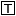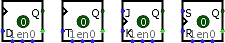#D/T/J-K/S-R Flip-Flop

 Library: Memory Introduced: 2.0 Beta 1 Appearance:## Behavior

Each flip-flop stores a single bit of data, which is emitted through the Q output on the east side. Normally, the value can be controlled via the inputs to the west side. In particular, the value changes when the clock input, marked by a triangle on each flip-flop, rises from 0 to 1 (or otherwise as configured); on this rising edge, the value changes according to the table below.

D Flip-Flop T Flip-Flop J-K Flip-Flop S-R Flip-Flop
DQ
00
11
TQ
0Q
1Q'
JKQ
00 Q
01 0
10 1
11 Q'
SRQ
00 Q
01 0
10 1
11 ??

Another way of describing the different behavior of the flip-flops is in English text.

• D Flip-Flop: When the clock triggers, the value remembered by the flip-flop becomes the value of the D input (Data) at that instant.

• T Flip-Flop: When the clock triggers, the value remembered by the flip-flop either toggles or remains the same depending on whether the T input (Toggle) is 1 or 0.

• J-K Flip-Flop: When the clock triggers, the value remembered by the flip-flop toggles if the J and K inputs are both 1 and the value remains the same if both are 0; if they are different, then the value becomes 1 if the J (Jump) input is 1 and 0 if the K (Kill) input is 1.

• S-R Flip-Flop: When the clock triggers, the value remembered by the flip-flop remains unchanged if R and S are both 0, becomes 0 if the R input (Reset) is 1, and becomes 1 if the S input (Set) is 1. The behavior in unspecified if both inputs are 1. (In Logisim, the value in the flip-flop remains unchanged.)

By default, the clock triggers on a rising edge — that is, when the clock input changes from 0 to 1. However, the Trigger attribute allows this to change to a falling edge (when the clock input changes from 1 to 0), a high level (for the duration that the clock input is 1), or a low level (for the duration that the clock input is 0). The level-trigger options are unavailable for the T and J-K flip-flops, because a flip-flop behaves unpredictably when told to toggle for an indeterminate amount of time.

## Pins

West edge, marked by triangle (input, bit width 1)
Clock input: At the instant that this input value switches from 0 to 1 (the rising edge), the value will be updated according to the other inputs on the west edge. As long as this remains 0 or 1, the other inputs on the west edge have no effect.
West edge, other labeled pin(s) (input(s), bit width 1)
These inputs control how the flip-flop's value changes during the rising edge of the clock. Their exact behavior depends on the flip-flop; the above tables summarize their behavior.
East edge, labeled Q, north end (output, bit width 1)
Outputs the value currently stored by the flip-flop.
East edge, south end (output, bit width 1)
Outputs the complement of the value currently stored by the flip-flop.
South edge, east end (input, bit width 1)
Asynchronous reset: When 0 or undefined, this input has no effect. As long as it is 1, the flip-flop's value is pinned to 0. This occurs asynchronously - that is, without regard to the current clock input value. As long as this is 1, the other inputs have no effect.
South edge, center end (input, bit width 1)
Enable: When this is 0, clock triggers are ignored. The current bit continues to appear on the output. The clock triggers are enabled when this input is 1 or undefined.
South edge, west end (input, bit width 1)
Asynchronous set: When 1 or undefined, this input has no effect. When 1, the flip-flop's value is pinned to 1. This occurs asynchronously - that is, without regard to the current clock input value. As long as this input is 1, the other inputs have no effect, except for the asynchronous reset input, which has priority.

## Attributes

Trigger
Configures how the clock input is interpreted. The value rising edge indicates that the flip-flop should update its value at the instant when the clock rises from 0 to 1. The falling edge value indicates that it should update at the instant the clock falls from 1 to 0. The high level value indicates that the flip-flop should update continuously whenever the clock input is 1. And the low level value indicates that it should update continuously when the clock input is 0. Note that the latter two options are unavailable for T and J-K flip-flops.
Label
The text within the label associated with the flip-flop.
Label Font
The font with which to render the label.

## Poke Tool Behavior

Clicking a flip-flop using the Poke Tool toggles the bit stored in the flip-flop, unless the asynchronous set/reset inputs currently pin the flip-flop's value.

## Text Tool Behavior

Allows the label associated with the component to be edited.

Back to Library Reference